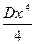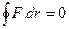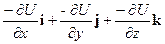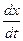# kinetic and potential energy worksheet

In this page we have kinetic and potential energy worksheet . Hope you like them and do not forget to like , social share and comment at the end of the page.
Question 1
A peculiar spring has a force law
F = -Dx3
which of the following is true
(a) potential energy at point x when U = 0 at x = 0 is(b) if a mass m is attached to the spring. Mass is displaced slightly, it will follow simple harmonic motion
(c)work has to be done on the spring in stretching it slowing from 0 to x
(d) none of the above
Question 2
An object of mass M slides downward along a plane inclined at angle θ .
The coefficient of friction is k
Find d(U + KE)/dt
(a) kmg2cosθ (sinθ - kcosθ)
(b) kmg2sinθ (sinθ - kcosθ)
(c) kmg2 (sinθ - kcosθ)
(d) none of the above
Question 3
What is true for gravitational force
(a) work done between the two points does not depend on the path travelled
(b) work done in a closed loop is zero
(c) W = - ΔU
(d) it is a conservative force
Question 4
An object moves on a inclined plane in upward direction at uniform velocity. Neglect frictional force. Which of the following is true
(a) gravitational force does negative work
(b) engine does positive work
(c) normal reaction force does not do any work
(d) none of the above

Question 5
If F is the conservative force, U is potential energy associated with this force and dr... is displacement. Which of the following is true
(a)(b) dU.=F.dr
(c) F(d) none of the above
Question 6
A body(m) is moving along positive x axis at t = 0, v = v0 and x = 0
It is subjected to retarded force
F = -2x
Find the x coordinate, whereis 0
(a) x = v0 √(m/2)
(b) x = v0 √(m)
(c) x = v0 √(2m)
(d) none of the above
Question 7
A particle moves from rest at point P1 on the surface of the smooth circular cylinder of radius R
Find the angle it will leave the cylinder
(a) θ = sin-12/3
(b) θ = cos-11/3
(c) θ = cos-12/3
(d) none of the above
Question 8
The kinetic energy of a particle moving along a circle of radius R depend on the distance covered S as
T = aS2
where a is constant
find the force acting on the particle as a function of S
(a) 2aS2/R
(b) 2aS √[1 + (S/R)2]
(c) 2aS √[1 - (S/R)2]
(d) none of the above
Question 9
A body is thrown vertically upward with a velocity v. It rise to some height and come back. find the mean power over all cycle
(a) mgv
(b) 0
(c) mgv2
(d) none of the above
1. (a), (c)
2. (a)
3. all
4. (a), (b), (c)
5. (a), (c)
6. (a)
7. (c)
8. (b)
9. (b)

Solutions